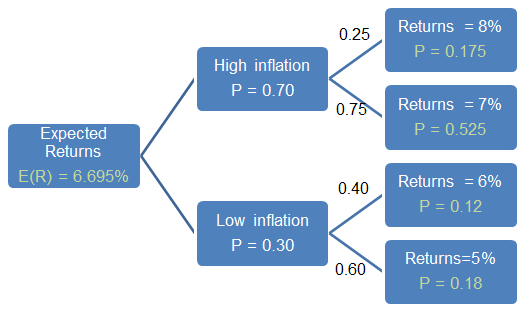# Conditional Expected Values

We can use the concept of conditional probabilities to arrive at the conditional expected values. Conditional expected values are conditional based on another event. For example, expected value of a random variable X given scenario S. A practical example would be to find the expected returns from a stock given rising inflation.

The total probability rule can be stated in terms of the expected values as follows:

E(X) = E(X | S1)P(S1) + E(X | S2)P(S2) + … + E(X | Sn)P(Sn)

Where the scenarios S1, S2, …Sn are mutually exclusive and exhaustive.

Example Using Tree Diagram

The following diagram shows the returns from a stock under different inflationary scenarios.Conditional Expected Value

The diagram shows that the probability of high inflation is 0.70 and the probability of low inflation is 0.30. Given high inflation, the probability of getting a return of 8% is 0.25 and probability of getting a return of 7% is 0.75. Given low inflation, the probability of getting a return of 6% is 0.40 and probability of getting a return of 5% is 0.60.

In the above tree diagram, the values in green are calculated values.

The joint probability of 8% return and high inflation is = 0.25*0.70 = 0.175

The joint probability of 7% return and high inflation is = 0.75*0.70 = 0.525

The joint probability of 6% return and low inflation is = 0.40*0.30 = 0.12

The joint probability of 5% return and low inflation is = 0.60*0.30 = 0.18

The expected return will be calculated as follows:

E(R) = 0.175*8%+0.525*7%+0.12*6%+0.18*5% = 6.695%

Get our Data Science for Finance Bundle for just $29$51.